﻿ Special Relativity: Resolving the Twin Paradox while Proving the Traveling Twin Cannot be YoungerInternational Journal of Theoretical and Mathematical Physics

p-ISSN: 2167-6844    e-ISSN: 2167-6852

2019;  9(2): 55-61

doi:10.5923/j.ijtmp.20190902.06### Special Relativity: Resolving the Twin Paradox while Proving the Traveling Twin Cannot be Younger

Manhar L. Shah

MVM Electronics, Inc., 3410 N. Harbor City Blvd. Suite E, Melbourne, FL, USA

Correspondence to: Manhar L. Shah , MVM Electronics, Inc., 3410 N. Harbor City Blvd. Suite E, Melbourne, FL, USA.
 Email: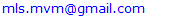Abstract

In special relativity, the “Twin Paradox” occurs when one twin makes a round trip journey. Lorentz transformations do not define which twin will be younger, only that they cannot be the same age upon return. Solutions that use discussions of inertial frames as well as accounting for acceleration and deceleration have been proposed to determine which twin is traveling. Over more than a century, many articles have been published to support the concept that the traveling twin will be younger upon return; however many of these arguments have flaws. This paper proposes a novel solution to resolving the “Twin Paradox” that requires that the traveling twin cannot return younger. While studying light propagation in the direction normal to the motion between two frames, it was discovered that the currently accepted principle of a younger traveling twin is in error. Additionally, it allows us to identify who is the traveler without ambiguity. The main argument against the currently accepted paradigm arises from this set-up which shows the apparent speed of light measured by the traveling twin, with the assumption that he will be younger, will be faster than the maximum permissible value c that is the speed of light in an inertial frame through a vacuum. That demonstrates a fundamental violation of the universally accepted limit on the speed of any entity. Further analysis shows that the traveling twin actually will be older as opposed to younger and will reverse the aging relation that is currently accepted.

Keywords: Special relativity, Twin paradox, Time dilation, Time travel, Clock paradox

Cite this paper: Manhar L. Shah , Special Relativity: Resolving the Twin Paradox while Proving the Traveling Twin Cannot be Younger, International Journal of Theoretical and Mathematical Physics, Vol. 9 No. 2, 2019, pp. 55-61. doi: 10.5923/j.ijtmp.20190902.06.

### 1. Introduction

The “Twin Paradox” [1-3] arises from the special relativity result that requires that two observers in relative motion to each other with velocity v observe the clock speed of the other as slower than his own. Therefore, if an observer makes a round trip journey and returns to the original position in a stationary frame after traveling some distance, then both the stationary and traveling twin would claim that his elapsed time is shorter than his twin. To establish the correct age of the twins some additional requirements must be met. A large number of papers have been written on this subject . Various explanations, theories and calculations are used to determine which twin is actually traveling and then to justify that the traveling twin will be younger upon return [5-9]. Papers also exist to justify an older traveling twin with invocation of the effect of acceleration  and no age difference with non-Lorenz transformation . The “Twin Paradox” being an integral part of special relativity,  its resolution is extremely important to fully embrace the theory.
Lorentz transformation  dictates that the twins cannot be the same age, but it doesn’t provide a compelling reason to choose whether the traveling or stationary twin will be younger. If one looks at Lorentz transformation in a casual manner then one presumes identical time dilation in the forward and return journeys of the traveling twin. Adding dilated times to both legs of the journey by the traveling twin seems to show that the traveling twin will be younger. On the other hand, if one calculates the time relation at the starting or reference point between two frames, it then appears that the stationary twin is younger. Since only one outcome can be true an additional constraint is required. Asymmetry between the stationary and traveling twins’ journeys arising from the traveling twin’s acceleration, deceleration, turn around and Doppler Effect eliminates the necessity that both twins age identically. However, non-symmetry of the situation considering those attributes is not sufficient to establish that the traveling twin will be younger. Effects of gravity and acceleration (general relativity) do not play any role in special relativity because we may consider only kinematics. Moreover, accounting for asymmetric attributes does not fully resolve the ambiguity about who is the traveler . The concept of the younger traveling twin is favoured by citing that the traveling frame can be reversed by frame switching and therefore the concept of time dilation will be the same in both directions. None of these explanations fully resolve the underlying paradox nor do they convincingly settle the question of which twin will be younger. Explanations that require frame switching by the traveling twin on his reverse journey fits the current concept of a younger traveling twin. This paper argues that such an assumption will produce a situation in which the measured speed of light by the traveling twin will exceed the maximum universally accepted speed of light c = 3x108 m/s. Lorentz transformation requires that the twins not be the same age, but no theoretical compulsion exists to accept the traveling twin to be younger. Therefore, if the only choice is that the traveling twin is younger or older, then we have to accept the traveling twin as older to satisfy the ultimate speed limit of any entity being c.
This paper proposes a new solution to the “Twin Paradox” using light propagation in a direction orthogonal to the motion. This novel approach is selected to avoid length contraction effect in that direction. It also elegantly explains why the traveling twin cannot be younger while only requiring use of the following widely accepted concepts: c = 3x108 m/s is the maximum possible speed of any entity and light travels in a straight line in an inertial frame. Additionally, the direction of light propagation in any direction other than the direction of motion changes in a frame if the frame velocity changes. In the course of this investigation it was revealed that light pulse propagation in a frame can also uniquely identify its designation as inertial or non-inertial. This identification is unlike those generated using acceleration, gravity, turn around or Doppler Effect which are shown to have some inconsistencies  that can make either twin the traveler.
A case showing the measured speed of light exceeds c if the traveling twin is younger is first presented. A discussion is presented in the next section to highlight deficiencies in explanations by several authors who support this concept. Propagation of light in a frame with changing velocity is presented and demonstrates how it establishes which twin is the traveler. Computations of light path lengths with condition of simultaneity are presented in which light propagates in a direction orthogonal to the motion of the frame. The computations show that in the traveling twin’s frame, light path length is g times the stationary twin’s frame light path length and not 1/g times as currently accepted by the traveling twin being younger. Here g is Lorentz contraction factor given as ,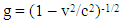(1)
Computations of light path lengths are given subsequently in which light pulse travels along the same direction as the twin’s motion.

### 2. A Set-up to Show why the Traveling Twin cannot be Younger

We consider a frame F that remains stationary and another coincident frame at the start of time designated as F’ that moves. An observer A is at the origin in frame F and another observer A’ is at the origin in frame F’. Both observers synchronize time and set time t = t’ = 0. Frame F’ travels with a velocity V in z direction in F to a distance L and returns with the same velocity to the original position in frame F. Figure 1 graphically shows the travel taken by A’. The distance L in F is marked as Z1. A’ travels with equal velocity, V, in each direction so the Lorenz contraction factor g is the same for each direction.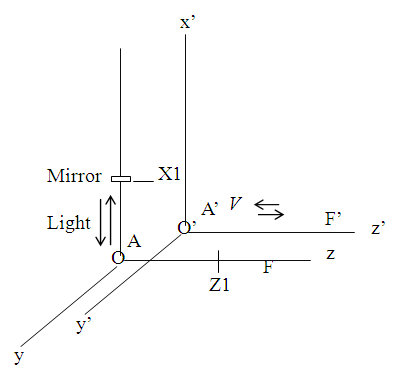Figure 1. Stationary frame F and round trip traveling frame F’. A mirror stationed at a distance LC/V marked X1 in F frame to reflect light sent from the origin
A mirror is placed in frame F along the x-axis (which is normal to the z-axis along which motion takes place) at distance X1 = Lc/V (this distance can be larger than Lc/V but we selected this value to simplify calculations) to reflect a light pulse transmitted from the origin of F back to the same origin. The experiment is set-up with precision (although not required if pulse length is sufficiently long but very much shorter than X1/c) so that the reflected light pulse arrives at the origin of F as well as A coincides with A’. If A’ is not in motion but stationary with A before the start and after the end of its journey then X1 can be slightly longer than Lc/V and A’ will receive the signal after its arrival at the origin. At t = t’ = 0 a light pulse is sent towards the mirror. A’ immediately departs for a round trip journey along z-axis with velocity V to a distance L in frame F. As stated before the forward and return journeys take place with the same velocity V. A’ returns to the origin and coincides with A’ after the journey. Since Lorenz transformation has x = x’, length contraction along the x-axis is not involved.
The round trip travel time, Tl, for light is equal to 2L/c. The round trip travel time by A’ as observed by A is, Ta, and equals to 2L/V. The round trip time observed by A’ is Tt. According to the currently accepted twin paradox concept Tt is smaller than Ta and equal to Ta/g. Nothing unusual is noticed by A when it receives the light pulse reflected from the mirror and calculates the speed of light. A receives the light pulse at the expected time 2L/V using light speed c. On the other hand, A’ would observe that it received the same pulse in time 2L/gV if the current concept of the twin paradox is used.
For measurements along the z-axis, length contraction occurs but here measurements of A’ are along the x-axis and length contraction is not involved. If light path length in F’ frame was shorter (by a factor g) then the velocity of light would be correct. But no possibility exists for finding a light path length shorter than 2L/V in F’ frame because light propagates along the x-axis in F frame and the Lorentz transformation has x’ = x. If light was propagating in a direction away from the x-axis then one can find the light path length in F’ frame shorter than the light path length in F frame. If A’ computes the speed of light using the shortest path from origin to the mirror then it computes the speed of light as,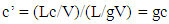(2)
Thus A’ computes that speed of light c’ is greater than c because g > 1 for any speed V > 0. Light path length in any direction other than x’ would produce a speed of light greater than gc. In this case, the length contraction for A’ as well as knowledge of light path in F’ frame are not involved. We can clearly conclude that the prevailing concept of the traveling twin being younger is in error. In this case, as dictated by Lorentz transformation, we have to accept the traveling twin as older and Tt = gTa.
As discussed in the next section, in stationary frame F, light travels in a straight line, but in F’ frame, which moves back and forth, light may not travel in a straight line from start to finish if light is propagating in a direction away from the direction of motion. In fact the stellar aberration data provides evidence in support of this. In the broader universe or a star’s stationary frame, the earth frame is moving and reverses velocity direction (due to the elliptical orbit of earth). Light propagation direction changes in our moving earth frame with this velocity reversal and results in the change of a star’s altitude known as stellar aberration. In the twin case, calculated speed of light equal to c is possible if light didn’t travel in a straight line in F’ frame. In that case light path length will be longer. The twin paradox shows we can have Tt either equal to Tag or Ta/g. Since Tt = Ta/g is not acceptable then it should be equal to Tag. Therefore, it is possible for the traveling twin to be older but not younger.
We further examine the situation in which F’ is not stationary but traveling at velocity V even when A’ coincides with A at the origin. We use the same frames as before with the mirror in F frame. At t = t’ = 0, A and A’ coincide when the pulse is initiated. Therefore, A’ knows the start time of the pulse. When A’ is passing the origin and coincides with A during its return trip, at that time the reflected pulse arrives at the origin and both A and A’ record the pulse arrival time according to their respective clocks. If A’ computes the velocity of light with light traveling in a straight line from the origin to the mirror and back, it computes the velocity of light equal to gc. This violates a fundamental principle: light cannot travel at a speed faster than c. Even if light travelled in a straight line in F’, the clock of A’ cannot show a time less than 2L/V, and definitely not close to 2L/Vg, implying that the traveling twin will be younger is in error.
It wouldn’t matter whether A or A’ initiated the pulse, because A and A’ were coincident at the initiation and receipt of the pulse. Therefore, both should register the events. It should be emphasized that in this case length contraction for A’ along z’-axis due to velocity V as well as the question of who emitted the light signal has no effect on the outcome. This case produces a contradictory result for the velocity of light if the currently accepted twin paradox concept in which the traveling twin younger is used. However, F’ being a non-inertial frame in which velocity reversal occurs, light may propagate in piecewise straight line directions (in F’ frame), allowing a longer path length for light to travel. Then the twin associated with A’ may be older than the twin associated with A. The apparent velocity of light less than c is acceptable for A’, however, as shown in a later section, the light path length in F’ frame is calculated to be Tt = Tag and light velocity equal to c is obtained.
If the mirror was placed on the z-axis at the same distance as X1 and light travelled along the z-axis, one could get the correct velocity of light for A’ if length contraction factor g is accepted in both forward and returned journeys. The catch is that the reflection from the mirror amounts to frame change. Then the situation erroneously predicts the traveling twin as younger. Frame change also occurs in the situation for light traveling along x-axis when the mirror is used. Clarification is needed to accept the result of this situation to prove that the traveling twin is younger concept is wrong.
In the case for light traveling along the x-axis, concern for frame switching can be avoided by placing two observers B and B’ in F and F’, respectively at distance 2X1 on the x and x’-axis, respectively. At t = t’ = 0 in F and F’ frames, A’ will coincide with A and B’ will coincide with B. Therefore, when A registers the start of light pulse, A’ will also register the same and similarly, when B registers the received pulse, B’ will also register it. Since, A and A’, and B and B’ coincide, they register the event as simultaneous. Thus, the outcome is the same as what we have previously obtained with the mirror on x-axis.

### 3. Deficiency of Earlier Explanations

An excellent discussion on the deficiencies in explaining the “Twin Paradox” in different situations is given by Unnikrishnan . An extensive bibliography, history and comparison of approach by many authors on the clock paradox are provided by Shuler . The discussion here focuses further highlighting those deficiencies. Many authors after reviewing extensive publications on the “Twin Paradox” have condensed the results in book form. Therefore, explanations of the traveling twin as younger concept from a few well-known books are used for discussion here.
As each twin can claim to be younger than the other, non-symmetry condition of travel is correctly applied to rule out the requirement of equal age for the twins. In most of these explanations, time dilation is assumed during the twin’s return journey similar to what occurs during his forward journey. This erroneously compels one to accept the traveling twin as younger. In the dominant explanations, discussion is inadequate to prove that the traveling twin as older violates basic principles or that the traveling twin as younger does not violate the same principles.
As discussed in , first the author shows correctly how the symmetry is broken. Then the author sites example of time spent by the traveling and stationary twin. The author uses one contraction factor or time warp factor over the entire journey for computing time for the traveling twin which is incorrect. When twin A’ starts the return journey he is no longer at the origin or the reference point, therefore the clock of the traveling twin may not follow the same rule as during the forward journey. The next section of this paper, in which a single reference light ray is used to obtain the space-time relations between twins, clearly shows that elapsed time for the traveling one, is greater, making him older.
In  the author explains the paradox by two methods. In the first method he explains in a manner similar to  with the same argument that during both the forward and return journey the traveling twin sees the length of his travel contracted by the same factor g. He correctly points out that the event 2, which is reversing the twin’s velocity, takes place at a far distance from the reference point. However, he considers that as a change of frames and treats the reverse journey the same as the forward journey. If frame switching were not used, then the velocity reversal would require –V in Lorentz transformation and only the origin can be used for the reference. As a result he would get t’ = gt and not t’ = t/g for the total journey.
In the second method, the author of  considers Doppler Effect and counts the number of pulses during travel. The author refers to a table in which he already has assumed the return time for the traveler as L/gV and obtains t’ = t/g. With such a prior assumption, the result obtained cannot be taken as correct. If the author had assumed the return time different he would have gotten some other value for t’. Further, the author invokes Hermann Bondi’s work to show word lines of the traveler during forward and return journeys. The integration is taken along two different paths implying two different frames. As pointed out, if frame switching is applied t’ = t/g will result but that will violate the limit of light speed. The question is “should we allow this limit to be violated or accept the traveling twin as older?”
While asymmetry of travel between twins and Lorentz transformation show one or the other twin has to be younger after a round trip journey,  presents an argument to show the twins should age equally. The definition of velocity in the paper’s eq. 1 is incorrect. Additionally, the authors don’t use Lorentz transformation but develop their own. With the use of an unproven concept, the conclusion drawn by the authors is unacceptable.

### 4. Propagation of Light Ray in a Frame with Changing Velocity

Before discussing and computing light path lengths in stationary and traveling frames some discussion on light propagation in a frame with changing velocity is essential. We have used the well-known facts that the ultimate speed limit is c and light propagates in a straight line with velocity c in an inertial frame. Another observation can be added that the same light pulse manifests in all frames inertial or non-inertial and all frames sense the same energy. This means a light pulse traces out simultaneity paths in stationary and moving inertial frame as well as non-inertial frames. The direction of propagation of a light pulse ray in an inertial frame is constant so if another frame changes velocity then the ray trace in this non-inertial frame has to change direction. It is particularly apparent when the light ray direction is away from the direction of motion. Change of ray direction with changing speed of the frame is also supported by the experimental results of stellar aberration. This makes it obvious for both traveling and stationary twins to conclude who is the traveler by detecting the direction of light ray propagation in each frame during velocity change.
The above result also shows that though a frame cannot measure its absolute velocity it can measure velocity changes using light ray pulses. This firmly establishes the identity of the traveling twin and resolves the “Twin Paradox.” There appears to be no case in which the stationary twin can be construed as traveling if ray trace is used for determination. This result offers a novel and rigorous procedure compared with earlier procedures, for establishing the asymmetry in Twin Paradox. Change in direction of ray propagation in a frame with a changing velocity complicates light path length calculations due to involvement of length contraction. For that reason we have selected a ray in a stationary frame along the x-axis for light path length calculations in the next section.

### 5. Computation of Light Path Length and Elapsed Time in F’ for Round Trip Journey

Light path length and hence the elapsed time in a stationary and a moving frame can be obtained with the condition of simultaneity using the theories of special relativity and Lorentz transformation. This condition allows tracing of light paths in stationary and moving frames. We use stationary frame F and moving frame F’ with the same notations as used earlier for Figure 1 and the moving frame traveling in z-direction. In order for an arbitrary point in the stationary frame to coincide with a point in a moving frame, the points need to have x = x’ and y = y’. Special relativity shows that the motion of an inertial frame effects the relation between two frames for time and length along the direction of motion (z-axis) only. The space-time relation between two frames is through Lorentz transformation and in many cases simply with contraction or time warp factor g which depends upon the relative velocity between frames. Special relativity and Lorentz transformation also show that the same time prevails in the xy (or x’y’) plane for a given value of z (or z’). Therefore, g can be obtained by computing light path length from the origin to some selected simultaneity points along x and x’ axes. This is done by thought process of sending a light pulse from the reference point (origin) towards a known simultaneity point in one frame and tracing the light pulse path to that point in each frame as motion takes place. If the light path has a component along the z-axis then length contraction would be applicable to light path length according to Lorentz transformation. For that reason, light path in the stationary frame is selected along the x-axis for simplicity. This procedure is similar to one as shown in . As stated in the previous section, light travels in a straight line with speed c in an inertial frame. F’ frame goes through acceleration, deceleration and course reversal as it makes a round trip journey which causes light to travel in piece-wise straight lines in F’. This effect has been verified by the observation of stellar aberration.
To obtaining the light path lengths we use frames F and F’ with the same notations as in Figure 1. Instead of using a mirror as was done in Figure 1, two additional observers B and B’ as mentioned before are used to compute light path lengths for the round trip journey by A’. As discussed in the previous paragraph, establishment of simultaneity of A and A’ after return is equivalent to simultaneity of B and B’ after return. One selects known points in F and F’ frames that would receive simultaneously a light pulse transmitted from the origin. In the twins’ case, known simultaneity points are equivalent to the origin itself as A’ returns to A or we could alternatively select B and B’. A light pulse transmitted from the origin has to reach B if we want to find the light path length. With light propagating in a straight line in the stationary F frame, the x-axis at the origin is the only possibility for light propagation. No other choice of simultaneity points exists for this ray. The currently accepted “traveling twin as younger” concept would amount to erroneously using light propagation from A’ to B’ in a straight line, contracting distance between A’ to B’ by factor g and frame switching. In contrast straight line ray propagation from A’ to B’ is not possible in F’ which goes for a round trip journey.
To obtain the light path lengths and elapsed times for a general case we use velocity V1 for time T1 during the forward journey and velocity V2 for time T2 during the return journey for A’. Figure 2 depicts the relevant notations and condition for this thought process for the forward journey. At t = t’ = 0 a light pulse is sent towards B. As A’ travels to the distance Z1 = V1T1, the light pulse will travel from the origin to distance X1 equal to cT1 along the x-axis and A’ will reach to the destination point. During the return journey of A’, the light pulse will travel distance X2 equal to cT2 from X1 along x-axis in F as A’ coincides with A’ and B coincides with B’. As the light pulse propagates along the x-axis in F during the forward journey, the light path traced out in F’ is a straight line as shown by the thick line in Figure 2 and as seen in F. The simultaneity point R is the point when the forward journey ends. This point has the coordinate (X1, 0) in F and (X1’, Z1’) in F’. The light path length in F is from point (0, 0) to (X1, 0) and from (0, 0) to (X1’, Z1’) in F’. The light path length in F is equal to X1= cT1. Light path length in Figure 2 for F’ is from (0, 0) to R with coordinate (X1’, Z1’) is not proper, as shown in F. The length contraction along the z-axis needs to be accounted for. The light path length X1’ = X1 is proper in both F and F’ because no length contraction occurs in this direction. Lorentz transformation provides Z1’ = g1Z1 where g1 is the Lorentz contraction factor for velocity V1. From the right angle triangle we obtain light path length L1’ in F’ as,(3)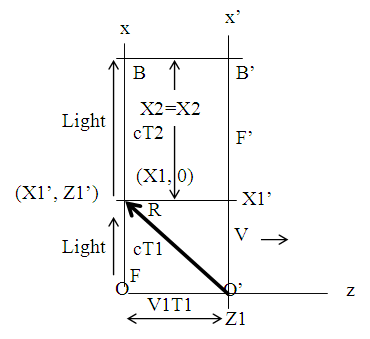Figure 2. Light path trace in F’ as seen by stationary frame F during the forward journey
We could have obtained (3) directly using Lorentz transformation. Substituting V1 and T1 for Z1’, X1’ and g1 in (3) we get T1’ = g1T1. Note that T1’ is the time in F’ at z = 0 not the time for A’.
The light path lengths from the simultaneity point R to the final simultaneity point where B’ coincides with B can be found in a similar manner. Adding the light path lengths of both segments of the journey we obtain total light path lengths and hence the elapsed times in F and F’ for the complete journey. The light path trace in F for the total round trip journey by A’ is shown in Figure 3 while the same light path trace in F’ is shown in Figure 4. The light path trace of A’ during the return journey is similar to the forward journey so we obtain T2’ = g2T2. At first glance one thinks that we should have V1T1 = V2T2, but this is not the case. Point R moves to a distance V2T2 from the origin in –z direction in F. Lorentz transformation will convert that distance in F to g2V2T2 in F’. The distance between R and the origin of F’ along the z-direction in F’ frame is g1V1T1. If A and A’ (and B and B’) are to coincide we need g1V1T1 = g2V2T2 and not V1T1 = V2T2. Combining times, we obtain total time T = T1 + T2 and T’ = T1’ + T2’ with relation T’ = g1T1 + g2T2. If T1 = T2 then we will have T’ = gT, so the traveling twin will be older and not younger. This result is opposite to the currently accepted concept of how the traveling twins ages relative to each other.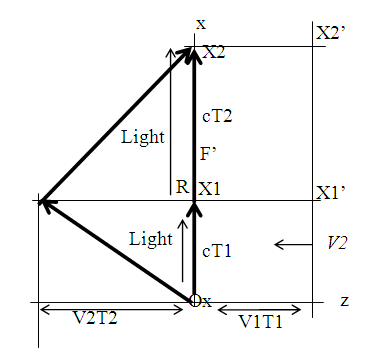Figure 3. Light path traces in F’ and F combined as seen in F for twin’s round trip journey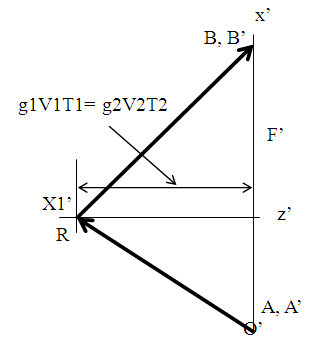Figure 4. Light path traces in moving frame F’ for twin’s round trip journey
It is natural to question what happens if the ray was selected along the z-axis instead of x-axis. One can justify younger traveling twin since length contraction occurs along the z-axis in this case. Figure 5 shows how A’ will move in F and how the light pulse will propagate in F and F’ for this case. The light path in F’ is shown with heavy lines. Of course, the light pulse will propagate in a straight line along the z-axis in both F and F’. At time t = t’ = 0 B and B’ must coincide due to positive velocity of F’ and length contraction as required by Lorentz transformation. Time of A’ at the end of the forward journey will be predicted by Lorentz transformation as T/2g where we have used T as total round trip time with the same velocity V for forward and reverse trip of A’. While A’ moves backward the light pulse will continue to the distance cT in F. When A’ arrives at the origin z = 0 it will be moving with velocity V in a negative direction. Lorentz transformation will give us cT length in F and cTg length in F’. A will coincide with A’ and B will coincide with B’ as found using Lorentz transformation. When A’ returns to the starting point each coincident point in F and F’ will coincide again. Converting the light path length in time we get T’ = gT as the elapsed time in F’. This confirms that the traveling twin is older.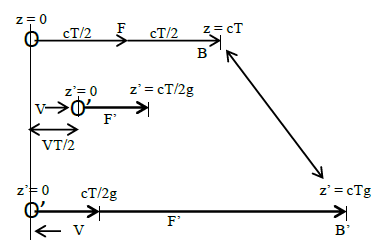Figure 5. Light path traces for light pulse propagation along z-axis for twin’s round trip journey

### 6. Conclusions

This paper presents a novel solution to the “Twins Paradox” using the observation of light propagation in a direction orthogonal to the direction of motion in order to determine the traveling twin. Propagation of a light ray in a frame with a changing velocity causes the ray direction to change. This effect can be used to measure velocity change of the frame and identify such frame as non-inertial and therefore identify which twin is traveling. Using this model and the broadly accepted concept that c is the upper limit of speed for an object in an inertial frame, the conclusion that the traveling twin must be older is obtained. This contradicts the widely held belief that the traveling twin in the thought experiment would return younger. The results can be used in other situations as well and suggest in general that time dilation is not possible with round trip travel in an inertial frame.